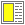# Exercise solution: The function outline-copy

Here is my first attempt (which is not correct):

```  (define (outline-copy x)
(cond ((pair? x)
(cons (outline-copy (car x)) (outline-copy (cdr x))))
(else '-)))```

The problem with this function is that it does not handle the empty list part correct.

Here is a better, and correct version:

```  (define (outline-copy-1 x)
(cond ((null? x) '())
((pair? x)
(cons (outline-copy-1 (car x)) (outline-copy-1 (cdr x))))
(else '-)))```

Notice how difficult it would be program the copying function without use of recursion. The reason is, of course, that we need recursive functions to process a recursive data structure.